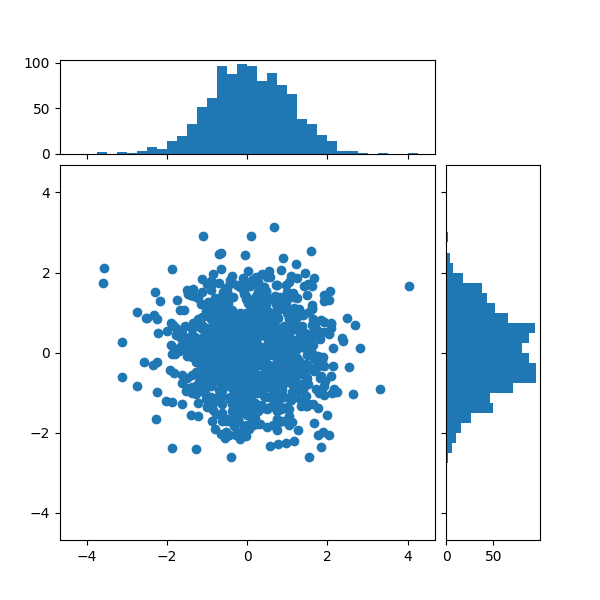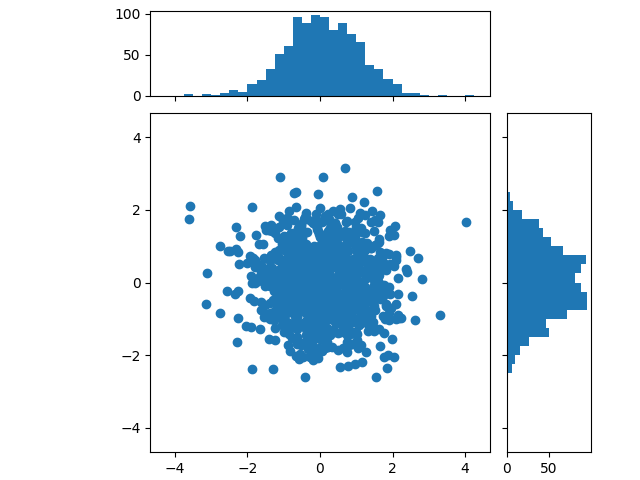# Scatter plot with histograms#

Show the marginal distributions of a scatter plot as histograms at the sides of the plot.

For a nice alignment of the main axes with the marginals, two options are shown below:

While Axes.inset_axes may be a bit more complex, it allows correct handling of main axes with a fixed aspect ratio.

An alternative method to produce a similar figure using the axes_grid1 toolkit is shown in the Scatter Histogram (Locatable Axes) example. Finally, it is also possible to position all axes in absolute coordinates using Figure.add_axes (not shown here).

Let us first define a function that takes x and y data as input, as well as three axes, the main axes for the scatter, and two marginal axes. It will then create the scatter and histograms inside the provided axes.

import numpy as np
import matplotlib.pyplot as plt

# Fixing random state for reproducibility
np.random.seed(19680801)

# some random data
x = np.random.randn(1000)
y = np.random.randn(1000)

def scatter_hist(x, y, ax, ax_histx, ax_histy):
# no labels
ax_histx.tick_params(axis="x", labelbottom=False)
ax_histy.tick_params(axis="y", labelleft=False)

# the scatter plot:
ax.scatter(x, y)

# now determine nice limits by hand:
binwidth = 0.25
xymax = max(np.max(np.abs(x)), np.max(np.abs(y)))
lim = (int(xymax/binwidth) + 1) * binwidth

bins = np.arange(-lim, lim + binwidth, binwidth)
ax_histx.hist(x, bins=bins)
ax_histy.hist(y, bins=bins, orientation='horizontal')


## Defining the axes positions using a gridspec#

We define a gridspec with unequal width- and height-ratios to achieve desired layout. Also see the Arranging multiple Axes in a Figure tutorial.

# Start with a square Figure.
fig = plt.figure(figsize=(6, 6))
# Add a gridspec with two rows and two columns and a ratio of 1 to 4 between
# the size of the marginal axes and the main axes in both directions.
# Also adjust the subplot parameters for a square plot.
gs = fig.add_gridspec(2, 2,  width_ratios=(4, 1), height_ratios=(1, 4),
left=0.1, right=0.9, bottom=0.1, top=0.9,
wspace=0.05, hspace=0.05)
# Create the Axes.
# Draw the scatter plot and marginals.
scatter_hist(x, y, ax, ax_histx, ax_histy)## Defining the axes positions using inset_axes#

inset_axes can be used to position marginals outside the main axes. The advantage of doing so is that the aspect ratio of the main axes can be fixed, and the marginals will always be drawn relative to the position of the axes.

# Create a Figure, which doesn't have to be square.
fig = plt.figure(layout='constrained')
# Create the main axes, leaving 25% of the figure space at the top and on the
# right to position marginals.
# The main axes' aspect can be fixed.
ax.set(aspect=1)
# Create marginal axes, which have 25% of the size of the main axes.  Note that
# the inset axes are positioned *outside* (on the right and the top) of the
# main axes, by specifying axes coordinates greater than 1.  Axes coordinates
# less than 0 would likewise specify positions on the left and the bottom of
# the main axes.
ax_histx = ax.inset_axes([0, 1.05, 1, 0.25], sharex=ax)
ax_histy = ax.inset_axes([1.05, 0, 0.25, 1], sharey=ax)
# Draw the scatter plot and marginals.
scatter_hist(x, y, ax, ax_histx, ax_histy)

plt.show()References

The use of the following functions, methods, classes and modules is shown in this example:

Total running time of the script: ( 0 minutes 1.285 seconds)

Gallery generated by Sphinx-Gallery